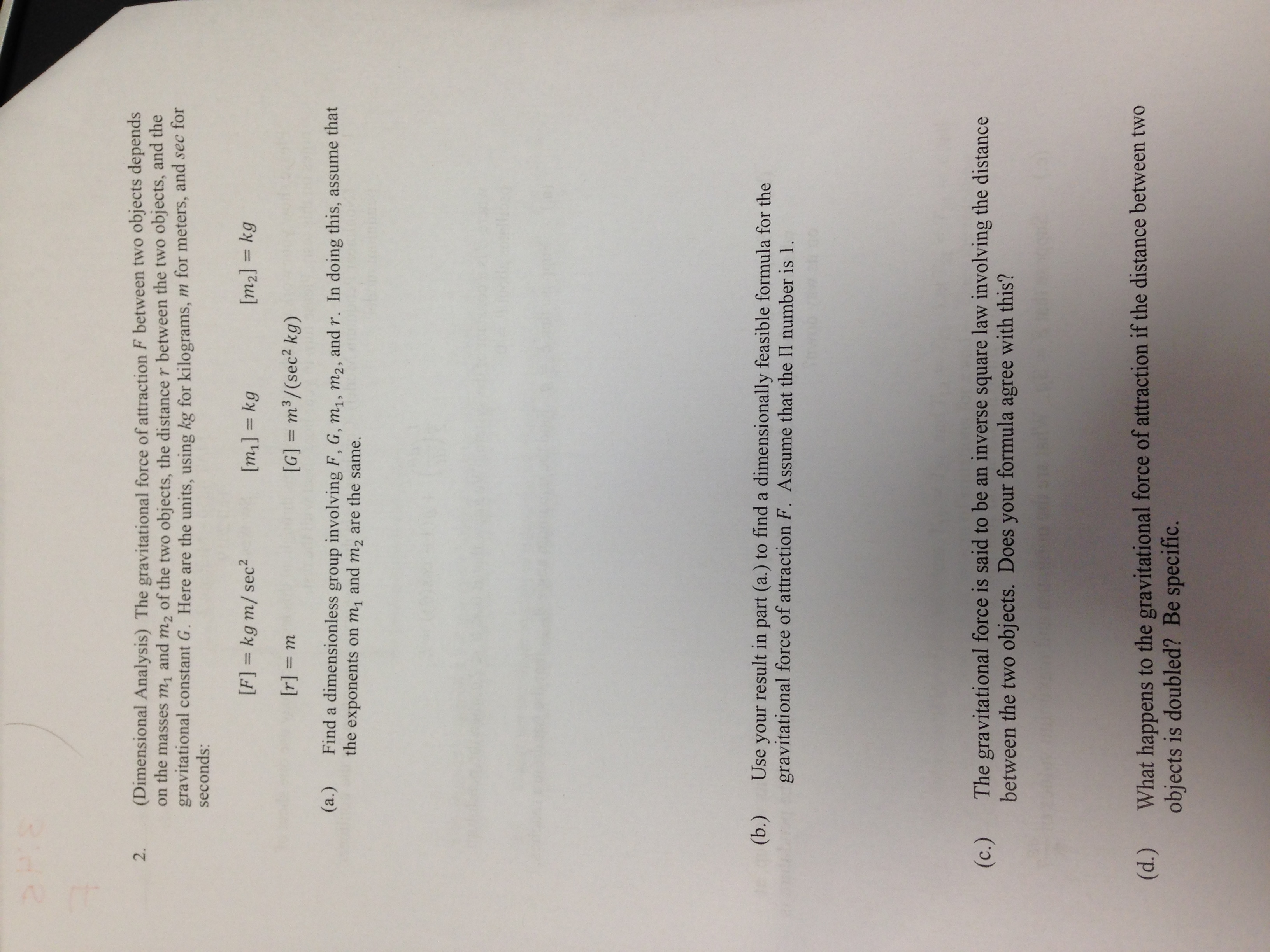# 2FI8(Dimensional Analysis) The gravitational force of attraction F between two objects dependson the masses m1 and m2 of the two objects, the distance r between the two objects, and thegravitational constant G. Here are the units, using kg for kilograms, m for meters, and sec forseconds:2.[F] = kg m/sec2[m2] = kg[m,] kg[r] m[G] m3/(sec2 kg)(а.)Find a dimensionless group involving F, G, m1, m2, and r. In doing this, assume thatthe exponents on mi and m2 are the same.(ь.)Use your result in part (a.) to find a dimensionally feasible formula for thegravitational force of attraction F. Assume that the II number is 1.The gravitational force is said to be an inverse square law involving the distancebetween the two objects. Does your formula(c.)with this?agreeWhat happens to the gravitational force of attraction if the distance between two(d.)objects is doubled? Be specific.

Question
25 viewshelp_outlineImage Transcriptionclose2FI8 (Dimensional Analysis) The gravitational force of attraction F between two objects depends on the masses m1 and m2 of the two objects, the distance r between the two objects, and the gravitational constant G. Here are the units, using kg for kilograms, m for meters, and sec for seconds: 2. [F] = kg m/sec2 [m2] = kg [m,] kg [r] m [G] m3/(sec2 kg) (а.) Find a dimensionless group involving F, G, m1, m2, and r. In doing this, assume that the exponents on mi and m2 are the same. (ь.) Use your result in part (a.) to find a dimensionally feasible formula for the gravitational force of attraction F. Assume that the II number is 1. The gravitational force is said to be an inverse square law involving the distance between the two objects. Does your formula (c.) with this? agree What happens to the gravitational force of attraction if the distance between two (d.) objects is doubled? Be specific. fullscreen
check_circle

Step 1

(a) The number of  dimensional variables are 5 namely, F, G, m1, m2 and r. The reference dimensions are M, L, T. Thus, according to the Buckingham Pi theorem, the number of non-dimensional variables are 5-3=2.

Step 2

Choose r and m2 as the repeating variables.

Find the first non-dimensional group

Step 3

Solve for the e...

### Want to see the full answer?

See Solution

#### Want to see this answer and more?

Solutions are written by subject experts who are available 24/7. Questions are typically answered within 1 hour.*

See Solution
*Response times may vary by subject and question.
Tagged in

### Science